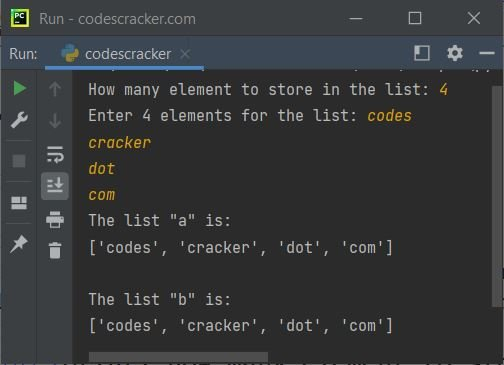# Python Program to Copy a List

• Copy a list using the "=" operator.
• Copy a list using the copy() function.
• Copy a list using list slicing.
• Copy a list using a loop and the append() function.

## Copy a list in Python using the = operator

The question is: write a Python program to copy a list. The program given below has its answer:

```a = [40, 50, 60]
print("The list \"a\" is:")
print(a)

b = a
print("\nThe list \"b\" is:")
print(b)```

The output from the aforementioned program, which demonstrates how to copy a list in Python, is as follows:

```The list "a" is:
[40, 50, 60]

The list "b" is:
[40, 50, 60]```

#### Copy a list entered by the user in Python

This is basically the same program as the previous program. The only difference is that this program allows the user to define the size of a list, along with its elements or items:

```print("How many element to store in the list: ", end="")
n = int(input())
print("Enter", n, "elements for the list: ", end="")
a = []
for i in range(n):
a.append(input())

print("The list \"a\" is:")
print(a)

b = a
print("\nThe list \"b\" is:")
print(b)```

The snapshot given below shows the sample run of the above Python program, with user input "4" as the number of elements to store in the list and "codes", "cracker", "dots", and "com" as the four elements:#### Points to be noted

Important: If a list is copied to another using the "=" operator, and if you change any list, then both lists will get changed. For example:

```a = [40, 50, 60]

b = a
print("The list \"b\" is:")
print(b)

a.append(70)
print("\nThe list \"b\" is:")
print(b)

b.append(80)
print("\nThe list \"a\" is:")
print(a)```

This program produces the following output:

```The list "b" is:
[40, 50, 60]

The list "b" is:
[40, 50, 60, 70]

The list "a" is:
[40, 50, 60, 70, 80]```

This happens because the new copied list is referencing the old list through which the new list was copied.

## Copy a list in Python without changing the original

To copy a list in Python without changing the original list, use the "copy()" function. Here is an example:

```a = [76, 87, 90]
print("The list \"a\" is:")
print(a)

b = a.copy()
print("\nThe list \"b\" is:")
print(b)

a.append(70)
print("\nNow the list \"a\" is:")
print(a)
print("\nThe list \"b\" is:")
print(b)

b.append(80)
print("\nThe list \"a\" is:")
print(a)
print("\nNow the list \"b\" is:")
print(b)```

The output would be:

```The list "a" is:
[76, 87, 90]

The list "b" is:
[76, 87, 90]

Now the list "a" is:
[76, 87, 90, 70]

The list "b" is:
[76, 87, 90]

The list "a" is:
[76, 87, 90, 70]

Now the list "b" is:
[76, 87, 90, 80]```

## Copy a list in Python using list slicing

Here is another program that shows how to copy a list in Python. This program copies a list using slicing.

```a = [76, 87, 90]

b = a[:]
print("The list \"b\" is:")
print(b)```

The output produced by the above Python code will exactly be:

```The list "b" is:
[76, 87, 90]```

After copying, whether you change the original or the copied list, the other list does not get affected.

## Copy a list in Python using a loop

This is the last program in this article, created to, of course, demonstrate how to copy a list, but using a loop (a "for" loop) this time:

```a = [76, 87, 90]

b = []
for x in a:
b.append(x)

print("The list \"b\" is:")
print(b)```

Or:

```a = [76, 87, 90]

b = []
for i in range(len(a)):
b.append(a[i])

print("The list \"b\" is:")
print(b)```

Both of the previous programs did the same job. Here is the same output produced by both of the programs given above:

```The list "b" is:
[76, 87, 90]```

Python Online Test

« Previous Program Next Program »